# Texas Go Math Grade 1 Lesson 7.4 Answer Key Use 10 to Subtract

Refer to our Texas Go Math Grade 1 Answer Key Pdf to score good marks in the exams. Test yourself by practicing the problems from Texas Go Math Grade 1 Lesson 7.4 Answer Key Use 10 to Subtract.

## Texas Go Math Grade 1 Lesson 7.4 Answer Key Use 10 to Subtract

Essential Question
Explanation:
By rounding to ten we can easily solve the subtract.

Explore
Useto show the problem.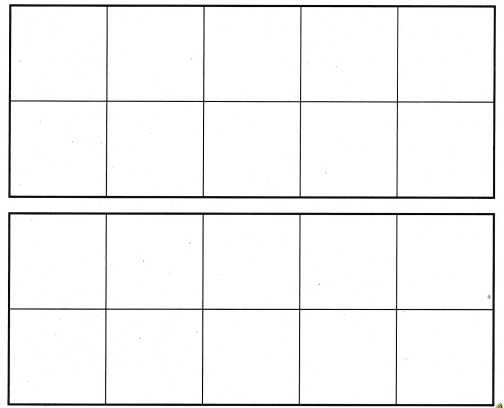For The Teacher

• Read the following problem. Austin puts 9 red counters in the first ten frame. Then he puts 1 yellow counter in the ten frame. How many more yellow counters does Austin need to make 15?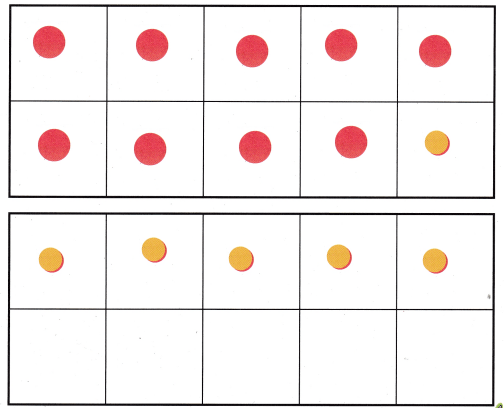9 are red and
5  more yellow counters that Austin need to make 15

Math Talk
Mathematical Processes
Explanation:
With the help of drawings we can easily subtract.

Model and DrawExplanation:Explanation:
The number is made to partners of 10
so make subtraction easy.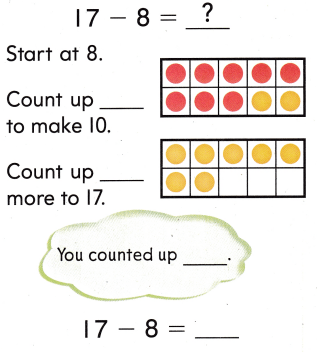Explanation:Explanation:
Started at 8 counted up to 10 in first ten frame
then again counted to make 17 in second ten frame

Share and Show

Use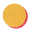and ten frames. Make a ten to subtract. Draw to show your work.

Question 1.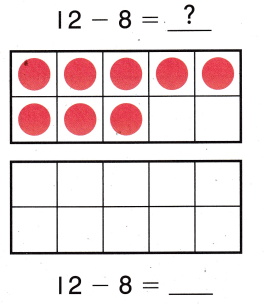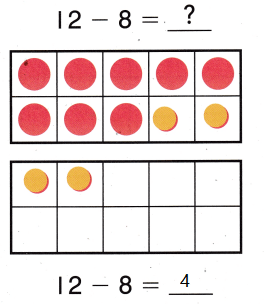Explanation:
Started at 8 counted up to 10 in first ten frame
then again counted to make 12 in second ten frame

Question 2.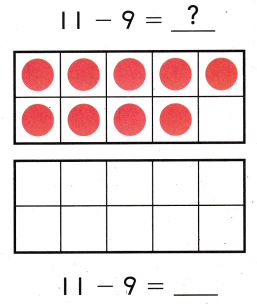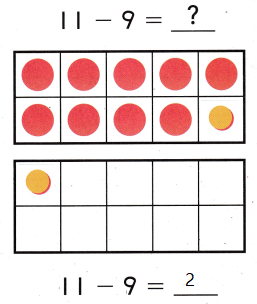Explanation:
Started at 9 counted up to 10 in first ten frame
then again counted to make 11 in second ten frame

Problem Solving

Useand ten frames. Make a ten to subtract. Draw to show your work.

Question 3.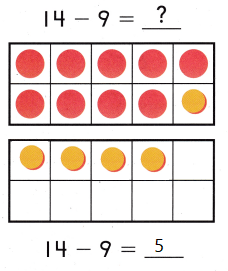Explanation:
Started at 9 counted up to 10 in first ten frame
then again counted to make 14 in second ten frame

Question 4.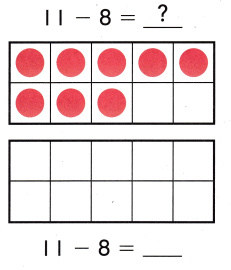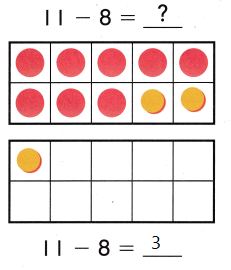Explanation:
Started at 8 counted up to 10 in first ten frame
then again counted to make 11 in second ten frame

Solve. Use the ten frames to make a ten to help you subtract.

Question 5.
________ stickers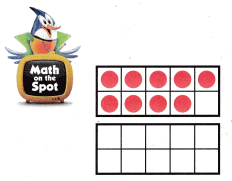16 stickers
Explanation:
John had some stickers. He gave 9 to April. Then he had 7.
9 + 7 = 16

Question 6.
does Alice have?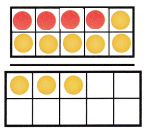Explanation:
that Alice have

Question 7.
Apply There are 14- flowers in the garden. 9 are red. The rest are yellow. How many flowers are yellow?
(A) 4
(B) 5
(C) 6
Explanation:
Apply There are 14- flowers in the garden. 9 are red.
14 – 9 = 5
The rest are yellow.
5 flowers are yellow

Question 8.
Daniel has 16 stickers. He gives 7 stickers to Bella. How many stickers does Daniel have now?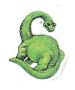(A) 5
(B) 7
(C) 9
Explanation:
Daniel has 16 stickers. He gives 7 stickers to Bella.
16 – 7 = 9
9 stickers that Daniel have now

Question 9.
Jayden walks II dogs each day. He has walked 6 dogs today. How many dogs are left to walk?
(A) 7
(B) 5
(C) 6Explanation:
Jayden walks II dogs each day. He has walked 6 dogs today.
11 – 6 = 5
5 dogs are left to walk

Question 10.
Texas Test Prep Which shows a way to make a ten to subtract?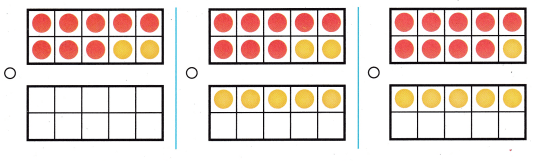Explanations:
Bubbled the 2 options to make a ten

Take Home Activity

• Ask your child how they make o ten to subtract eight or nine from a teen number.
19 – 9 = 10
So, in frame there will be 0 ten subtracted.

### Texas Go Math Grade 1 Lesson 7.4 Homework and Practice Answer Key

Make a ten to subtract. Draw to show your work.

Question 1.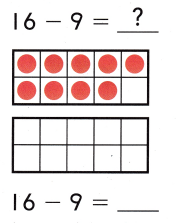Explanation:
Started at 9 counted up to 10 in first ten frame
then again counted to make 16 in second ten frame

Question 2.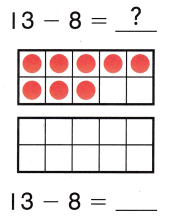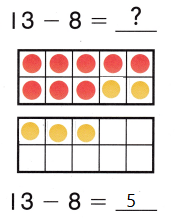Explanation:
Started at 8 counted up to 10 in first ten frame
then again counted to make 13 in second ten frame

Problem Solving

Solve. Use ten frames to make a ten to help you subtract.

Question 3.
On Monday, Owen had some video games. On Tuesday, he gave 8 to Anna. Then he had 6. How many video games did Owen have on Monday?
____ video games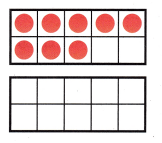14 video games
Explanation:
On Monday, Owen had some video games. On Tuesday, he gave 8 to Anna.
8 + 6 = 14
14 video games that Owen have on Monday

Texas Test Prep

Lesson Check

Question 4.
Noah invites 15 friends over. 8 are boys. The rest are girls. How many friends are girls?
(A) 8
(B) 7
(C) 9
Explanation:
Noah invites 15 friends over. 8 are boys.
15 – 8 = 7
The rest are girls.
7 friends are girls

Question 5.
Chloe has 12 pieces of chalk. She uses up 6 pieces. How many pieces of chalk does Chloe have now?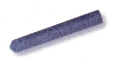(A) 5
(B) 7
(C) 6
Explanation:
Chloe has 12 pieces of chalk. She uses up 6 pieces.
12 – 6 = 6
6 pieces of chalk that Chloe have now

Question 6.
Which shows a way to make a ten to find 13 – 9?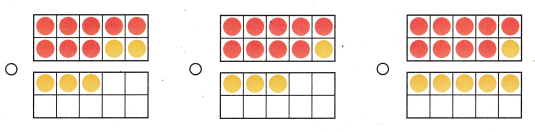Explanation:
13 – 9 = 4
started counting from 9
10 is counted in first frame
10 to 13 in second frame

Question 7.
Multi-Step Sydney drew 12 animal pictures. She gave 3 bird pictures to her mother. She gave 6 cat pictures to her father. How many pictures does she have left?
(A) 3
(B) 9
(C) 6